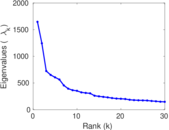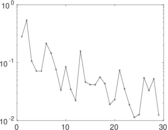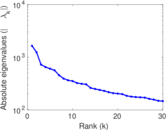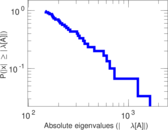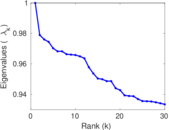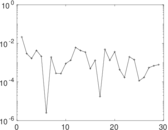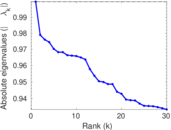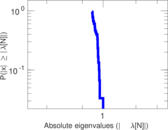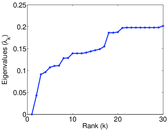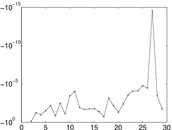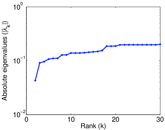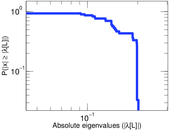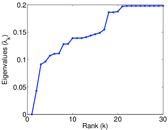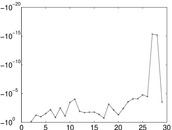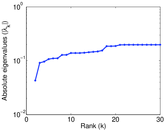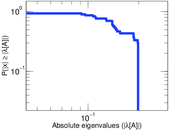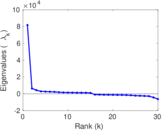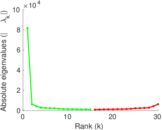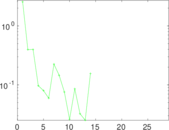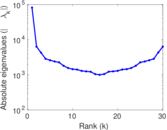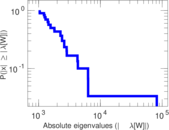# Wikipedia edits (ast)

This is the bipartite edit network of the Asturian Wikipedia. It contains users and pages from the Asturian Wikipedia, connected by edit events. Each edge represents an edit. The dataset includes the timestamp of each edit.

 Code `ast` Internal name `edit-astwiki` Name Wikipedia edits (ast) Data source http://dumps.wikimedia.org/ AvailabilityDataset is available for download Consistency checkDataset passed all tests Category Authorship network Dataset timestamp 2017-10-20 Node meaning User, article Edge meaning Edit Network formatBipartite, undirected Edge typeUnweighted, multiple edges Temporal dataEdges are annotated with timestamps

## Statistics

 Size n = 81,704 Left size n1 = 4,033 Right size n2 = 77,671 Volume m = 994,558 Unique edge count m̿ = 436,676 Wedge count s = 2,402,087,150 Claw count z = 17,633,789,427,562 Cross count x = 121,576,211,435,839,248 Square count q = 4,081,465,143 4-Tour count T4 = 42,261,423,196 Maximum degree dmax = 175,172 Maximum left degree d1max = 175,172 Maximum right degree d2max = 1,711 Average degree d = 24.345 4 Average left degree d1 = 246.605 Average right degree d2 = 12.804 8 Fill p = 0.001 394 03 Average edge multiplicity m̃ = 2.277 57 Size of LCC N = 80,307 Diameter δ = 12 50-Percentile effective diameter δ0.5 = 3.154 96 90-Percentile effective diameter δ0.9 = 3.866 24 Median distance δM = 4 Mean distance δm = 3.259 63 Gini coefficient G = 0.830 293 Balanced inequality ratio P = 0.165 777 Left balanced inequality ratio P1 = 0.033 717 5 Right balanced inequality ratio P2 = 0.246 428 Relative edge distribution entropy Her = 0.726 371 Power law exponent γ = 1.917 29 Tail power law exponent γt = 3.091 00 Tail power law exponent with p γ3 = 3.091 00 p-value p = 0.000 00 Left tail power law exponent with p γ3,1 = 1.721 00 Left p-value p1 = 0.000 00 Right tail power law exponent with p γ3,2 = 4.991 00 Right p-value p2 = 0.000 00 Degree assortativity ρ = −0.441 800 Degree assortativity p-value pρ = 0.000 00 Spectral norm α = 1,646.66 Algebraic connectivity a = 0.042 999 9 Spectral separation |λ1[A] / λ2[A]| = 1.324 35 Controllability C = 73,772 Relative controllability Cr = 0.909 564

## Plots

### Fruchterman–Reingold graph drawing### Degree distribution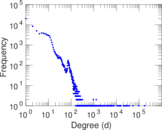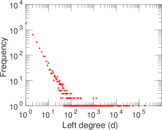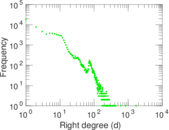### Cumulative degree distribution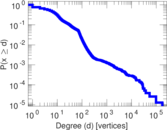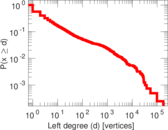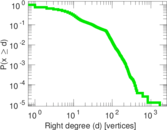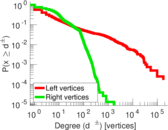### Lorenz curve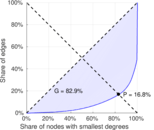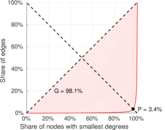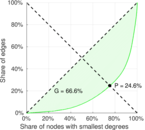### Spectral distribution of the adjacency matrix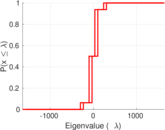### Spectral distribution of the normalized adjacency matrix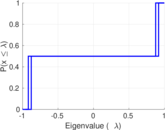### Spectral distribution of the Laplacian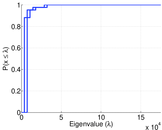### Spectral graph drawing based on the adjacency matrix### Spectral graph drawing based on the Laplacian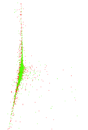### Spectral graph drawing based on the normalized adjacency matrix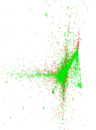### Degree assortativity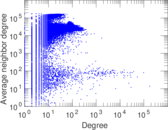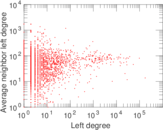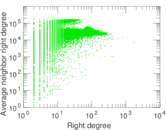### Zipf plot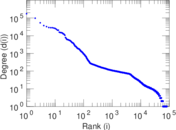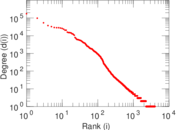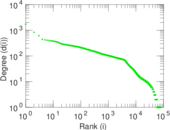### Hop distribution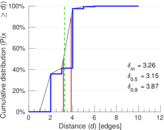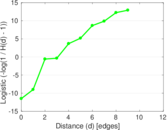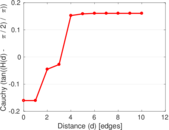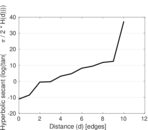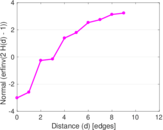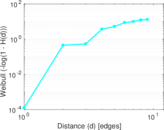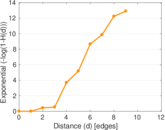### Double Laplacian graph drawing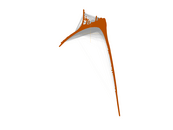### Delaunay graph drawing### Edge weight/multiplicity distribution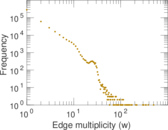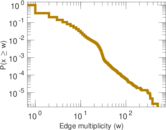### Temporal distribution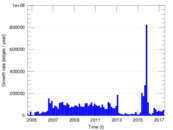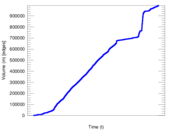### Temporal hop distribution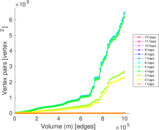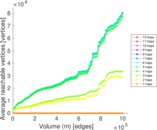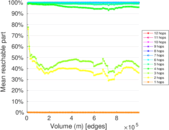### Diameter/density evolution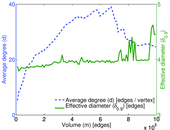### Matrix decompositions plots